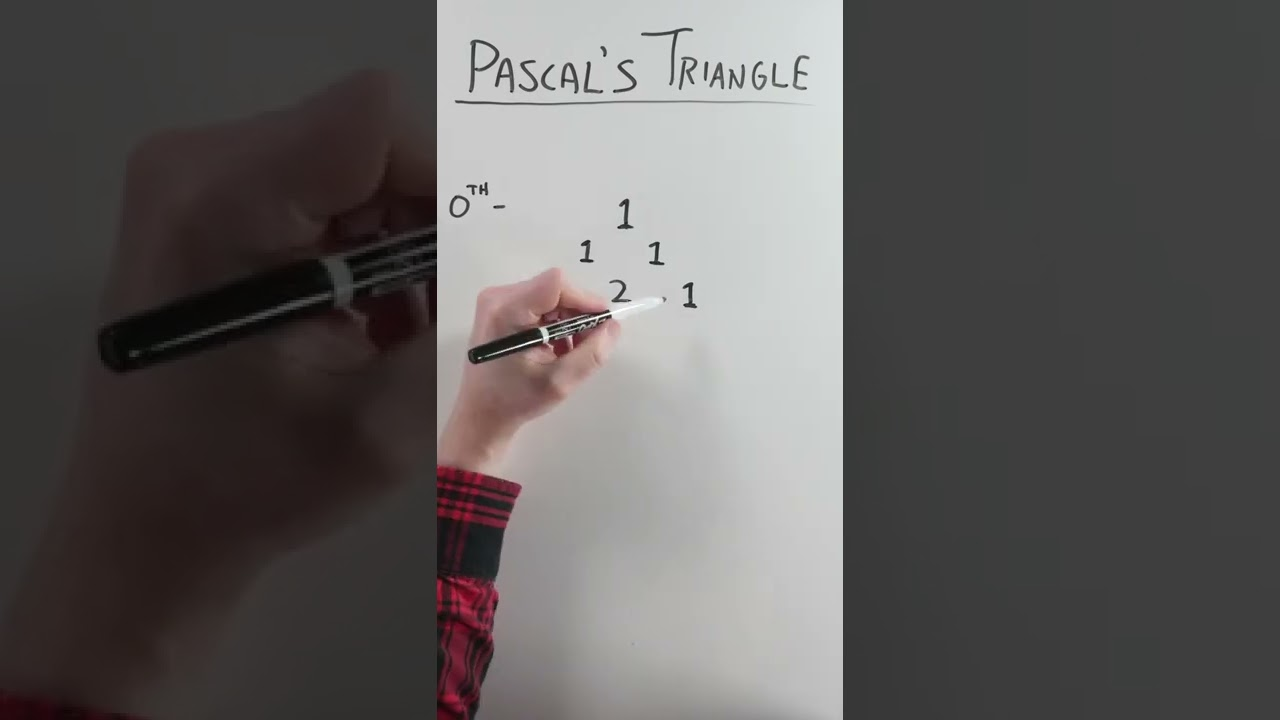Vsauce

# The Tip of Pascal’s Iceberg #shorts

Blaise Pascal may have published Traité du triangle arithmétique in 1665, but the world knew about his triangle centuries before. Scholars in India, Persia, China, and even Germany played with the possibilities of a pattern of binomial coefficients, but Pascal brought it to the broader world. Pascal’s Triangle is easy to construct by hand; just start with 1 and do the arithmetic.

As Pascal’s Triangle expands, it reveals mathematical patterns we value elsewhere, from the simple whole number line and the primacy of 1 to a succession of the powers of 2 and the digits of 11’s exponential sequence. And lurking within is a classic Fibonacci sequence in which each successive digit is the sum of its two predecessors.

But one of the most interesting elements of Pascal’s Triangle is the way it lays bare the possible outcomes of any series of simple coin flips. Like everything else in Pascal’s Triangle, it’s in there if you know where to look.

#shorts #vsauce #maths